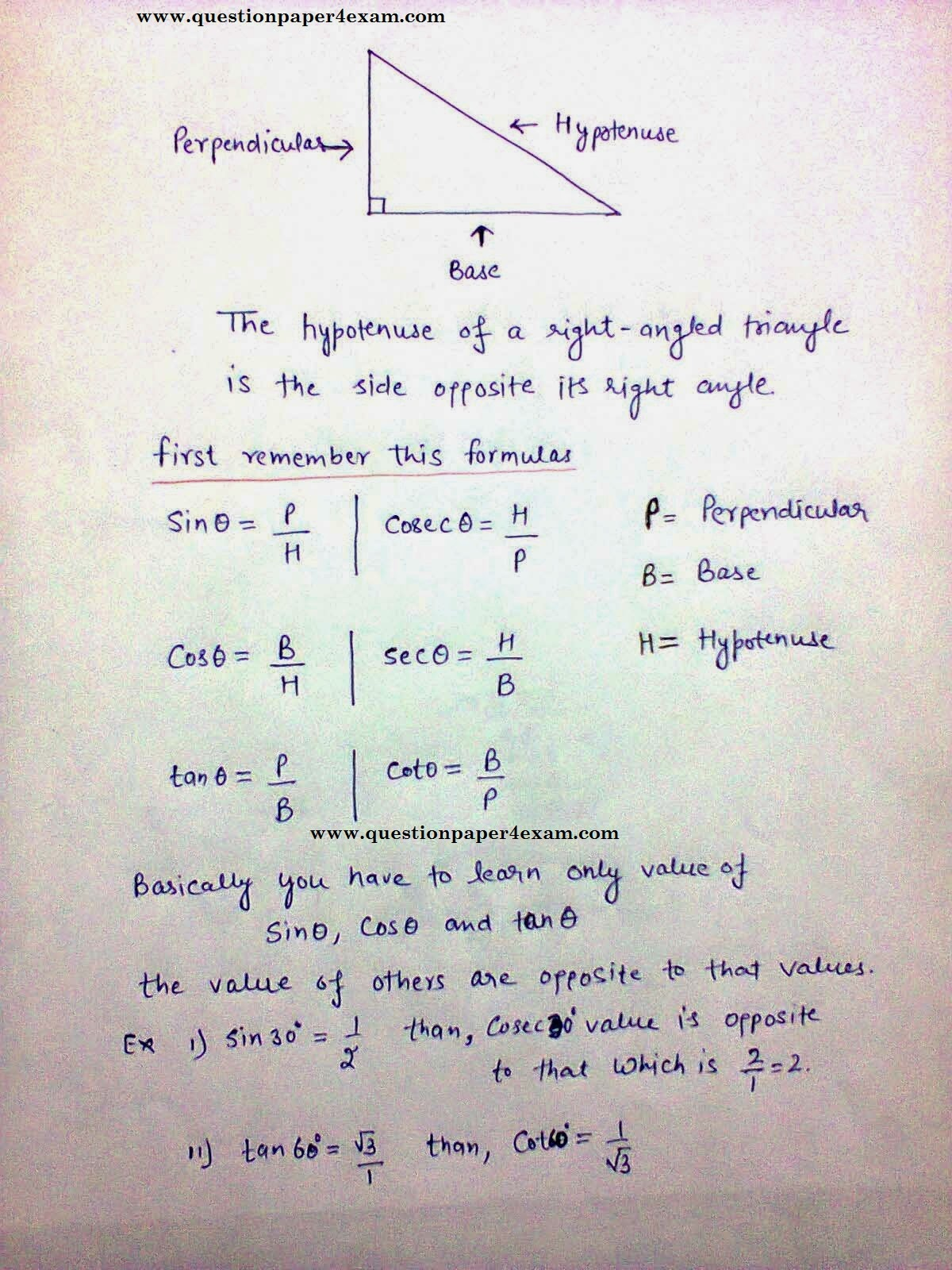# SSC EXAM TRIGONOMETRY BASIC CONCEPTS

## TRIGONOMETRY BASIC CONCEPT FOR SSC EXAM

DEFINITION OF TRIGONOMETRY :- trigonometry is the branch of mathematics that is concerned with calculating the angles of triangles or the lengths of their sides.

In this article we are provided the basic of trigonometry. Which are main topic in ssc exams. 4 –5 question are based on this topic in ssc exam and in the mainS of cgl 10 – 14 question are asked. So, it is the important one for preparation.

Basically we first provide basic concept and identities that are used to solving question based on trigonometry.

First of all you have to learn that following table.

in trigonometry there is no trick based question asked but if you learn the basic concept of trigonometry then you are easily solve within few seconds. Candidates find this is very tough topic to prepare for ssc some candidates leaves these chapter question in exam but this chapter is so easily prepare if you practice hard with this chapter by learn table and identities.

so, learn this following these helpful in solving question

 Ɵ SinƟ CosƟ TanƟ CosecƟ SecƟ CotƟ 0° 0 1 0 Not defined 1 Not  defined 30° 1 / 2 Ö3 / 2 1 / Ö3 2 2 / Ö3 Ö3 45° 1 / Ö2 1 / Ö2 1 Ö2 Ö2 1 60° Ö3 / 2 1 / 2 Ö3 2 / Ö3 2 1 / Ö3 90° 1 0 Not defined 1 Not defined 0
Some basic concept and  identities of trigonometrySome question with solution that asked in previous ssc paperSharing is caring:-

### 3 thoughts on “SSC EXAM TRIGONOMETRY BASIC CONCEPTS”

1.Sir,
thanku so much for helping us but please provide more questions on dese topics for practice test

2.